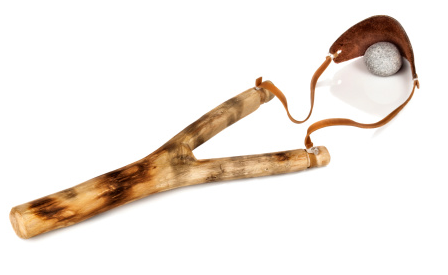## Conservation of Energy

A sling shot, which has a band stiffness of 125 N/m, is pulled back 0.15m. If the pellet/rock weighs 100 grams, what is the velocity of the pellet/rock immediately after release?Hint
Kinetic Energy:
$$KE=\frac{1}{2}mv^2$$$where $$m$$ is the mass, and $$v$$ is the velocity. Hint 2 Potential Energy: $$PE=\frac{1}{2}kx^2$$$
where $$k$$ is the spring constant/stiffness, and $$x$$ is the spring’s change in length from un-deformed to deformed.
This problem can be solved using the conversion of Potential Energy into kinetic. Because the sling shot acts like a spring, the potential energy is:
$$PE=\frac{1}{2}kx^2$$$where $$k$$ is the spring constant/stiffness, and $$x$$ is the spring’s change in length from un-deformed to deformed. $$PE=\frac{1}{2}\cdot 125\frac{N}{m}\cdot (0.15m)^{2}=1.4\:J$$$
Once released, the pellet/rock will be converted to Kinetic Energy:
$$KE=\frac{1}{2}mv^2$$$where $$m$$ is the mass, and $$v$$ is the velocity. $$KE=\frac{1}{2}(0.1kg)v^2=(0.05)v^2$$$
Assuming energy is conserved, then $$PE=KE$$ :
$$1.4\:\frac{kg\cdot m^2}{s^2}=(0.05\:kg)v^2$$$$$v^2=28\:\frac{m^2}{s^2}\Rightarrow v=\sqrt{28m^2/s^2}=5.3\:m/s$$$
5.3 m/s